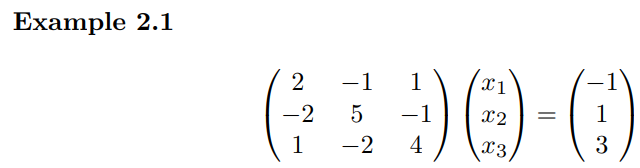# MATLAB code for successive over-relaxation (SOR) method

\$29

## Description

This MATLAB function receives a matrix A, a vector b, an initial starting vector x0, a real value ω, and a tolerance ε, and returns an approximate solution of the system Ax = b within the given tolerance together with the number of iterations.&gt;&gt; A = [2 -1 1; -2 5 -1; 1 -2 4] ; b = [-1;1;3] ;

&gt;&gt; x0 = [1.; 1.; 1.]; w = 1.25; Eps = 1e-8 ;

&gt;&gt; [x, Iters] = SOR(A, b, w, x0, Eps)

x =

-1.0000

0.0000

1.0000

Iters =

15A = [-5 1 -2; 1 6 3; 2 -1 -4] ; b = [13; 1; -1] ;
[x, Iters] = SOR(A, b, w, x0, Eps)

x =
-2.0000
1.0000
-1.0000
Iters =
33

## Reviews

There are no reviews yet.

SKU: 9MATSOR81 Category: